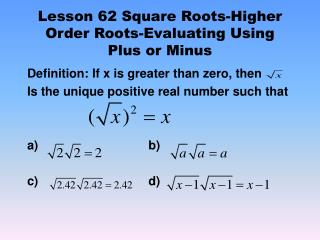DownloadDownload PresentationLesson 62 Square Roots-Higher Order Roots-Evaluating Using Plus or Minus

# Lesson 62 Square Roots-Higher Order Roots-Evaluating Using Plus or Minus

Télécharger la présentation## Lesson 62 Square Roots-Higher Order Roots-Evaluating Using Plus or Minus

- - - - - - - - - - - - - - - - - - - - - - - - - - - E N D - - - - - - - - - - - - - - - - - - - - - - - - - - -
##### Presentation Transcript

1. Lesson 62 Square Roots-Higher Order Roots-Evaluating Using Plus or Minus Definition: If x is greater than zero, then Is the unique positive real number such that a) b) c) d)

2. Ex 62.1 Use a calculator to determine to five decimal places Ex 62.2 Use a calculator to determine the two square roots of 10 to six decimal places. Ex 62.3 Without using a calculator, determine between what two consecutive integers the lies.

3. Lesson 62.B Ex 62.5 Without using a calculator, determine the following. Note that all answers are integers. a) b) c) Ex 62.6 Evaluate: